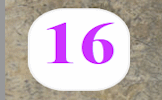# Visualise Percentages

0%

0

100% - This is the percent symbol.
Percent means 'out of 100'.

As 1 is one hundredth of 100, then 1% means 'one hundredth of'. To find 1% of a quantity you need to divide it by 100. So 1% of 800 is 8.

To find 2% of a quantity double 1% of it

To find 5% of a quantity halve 10% of it.

As 10 is one tenth of 100, then 10% means 'one tenth of'. To find 10% of a quantity you need to divide it by ten. So 10% of 800 is 80.

To find 20% of a quantity double 10% of it.

As 25 is one quarter of 100, then 25% means 'one quarter of'. To find 25% of a quantity you need to divide it by four. So 25% of 20 is 5.

Another way of finding 25% of a quantity is first finding 50% then dividing the result by 2.

As 33⅓ is one third of 100, then 33⅓% means 'one third of'. To find 33⅓% of a quantity you need to divide it by three. So 33⅓% of 30 is 10.

As 50 is half of 100, then 50% means half. To find 50% of a quantity you need to halve (or divide by two). So 50% of 6 is 3.

To find 52% of a quantity add 50% to 2% of that quantity

To find 66⅔% of a quantity double 33⅓% of it.

To find 75% of a quantity add together 50% and 25% of it.

To find 80% of a quantity subract 20% from that quantity.

To find 90% of a quantity subract 10% from that quantity.

If you need to use a calculator to check your working. See Calculator Workout skill 3.

### Commute

Did you know that if you are struggling to mentally work out 24% of 50 you can switch the numbers round and work out 50% of 24 instead. Finding 50% is very easy isn’t it? You will get the same answer.

Finding a percentage of a quantity is an example of a commutative calculation. Not all operations are commutative. Subtraction certainly isn’t as 10 minus one is not the same as one minus ten.

You can use this trick to improve your ability to do this type of calculation quickly if you find the switch makes it easier.

Practise with 12% of 50, 4% of 25 and 75% of 10.

#### Don't Shoot The SquareYou will need to be quick on the draw to shoot all of the numbers except the square numbers. So far this activity has been accessed 57806 times and 473 people have earned a Transum Trophy for completing it.

## Visualise Percentages

#### If you can picture in your mind what a percentage looks like you may be better able to preform mental calculations with that percentage.

This page is designed for teachers to use as a visual aid supporting the understanding of percentages

Click on a percentage button at the top of the page to see some of the more common percentages. Alternativelt click ony of the people in the grid to see the corresponding percentage.

Pupils can practice the skills of mentally calculating percentages by doing the online, self-marking exercise called PercentagesFor All:

©1997-2021 WWW.TRANSUM.ORG